Q&A - Ask Doubts and Get Answers

Sort by :
Clear All
Q

8  Water is pouring into a cubiodal reservoir at the rate of 60 litres per minute. If the volume of reservoir is   , find the number of hours it will take to fill the reservoir.Given that the water is pouring into a cuboidal reservoir at the rate of 60 litres per minute. Volume of reservoir is , then The number of hours it will take to fill the reservoir will be: As we know . Then  = 108,000 Litres ; Time taken to fill the tank will be: or   .

Q10. Find the values of the letters in each of the following and give reasons for the steps involved.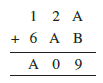The addition of A and B is giving a number whose ones digit is 9. The sum can only be 9 not 10 as a sum of two single digits cannot exceed 18. hence there will not be any carry for the next step  2 + A = 0  implies A = 8  2 + 8 = 10 and 1 is the carry for next step. 1 + 1 + 6 = A = 8  it satisfies hence A = 8 and B = 1 is the correct answer.

Q9. Find the values of the letters in each of the following and give reasons for the steps involved.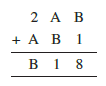The additiion of B and 1 is 8  is giving a number whose ones digit is 8. this means digit B is 7 .  B + 1 = 8 and no carry for next step. next step : Now, A + B = 1 => A + 7 which implies A = 4  A  + B = 11 and 1 is carry for next step  1 + 2 + A  = B 1 + 2 + 4 = 7  Hence A = 4 and B = 7 is correct answer.

Q8. Find the values of the letters in each of the following and give reasons for the steps involved.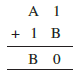The addition of 1 and B gives a number whose ones digit is 0. this is possible when digit B = 9 .  1 + B  = 10  and 1 is the Carry for the next step  Now, A + 1 + 1 = B => 9 Implies  A = 7. Hence A = 7 and B = 9 is Correct answer

Q7. Find the values of the letters in each of the following and give reasons for the steps involved.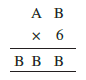The product of 6 & B gives a number whose unit digit is B again. possible value of B = 0. 2 , 4, 6 or 8  If B = o then our product will be zero. hence this value of B is not possible. If B = 2, then B x 6 = 12 . Carry for next step = 1. 6A + 1 = BB = 22 implies A = 21/6 = not any integer value hence this case is also not possible. If B = 6  then B *6 = 36 and 3 will be carry for next step. 6A +...

Q6.Find the values of the letters in each of the following and give reasons for the steps involved.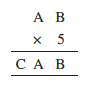Here , multiplication of B and 5 gives a number whose ones digit is B. this is possible when B = 0 or 5 let B = 5  B * 5 = 5 * 5 = 25 Carry = 2 5*A + 2 = CA  , This os possible only when A = 2 or 7 when A = 2 , 5*2 + 2 = 12 which implies C = 1 when A = 7  5*7 + 2 = 37 which implies C = 3   now  B = 0  B*5 = 0*5 = 0  Carry = 0 so  5 * A = CA which is possible when A = 0 or 5 Howerver A cannot...

Q5.Find the values of the letters in each of the following and give reasons for the steps involved. .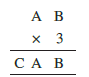Here multiplication of 3 and B gives  a number whose unit place digit is B . Possible value of B = 0 and 5 let B = 5  3 * A  + 1 = CA this is not possible for any value of A.  Hence B = 0 now  A * 3 = CA ( a number whose unit place digit is A itself when multiplied by 3) hence  possible value of A = 5 and 0  since AB is a two digit number A can not equal to 0. hence A = 5  A * 3 + 1 = CA 5 * 3...

Q4.Find the values of the letters in each of the following and give reasons for the steps involved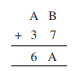There can be two cases 1. when the addition of unit place digit doesn't  produce Carry A + 3 = 6 A = 3 However, to get 3 in unit place of our answer our B has to be 6 and that would produce carry hence this case is not possible. 2. when the addition of unit place digit produces Carry A + 3 +1 = 6 A = 2 for getting 2 in unit place of answer we need the sum of unit digit of numbers = 12 B + 7 =...

Q3. Find the values of the letters in each of the following and give reasons for the steps involved.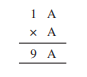Here first clue : we have A = a number which when multiplied by itself gives the same number in the unit digit.  possible numbers = 1 and 6  Second Clue: number when multiplied with 1 and added with the reminder of previous multiplication( A*A) = 9  both first and second clue implies that A = 6.

Q2. Find the values of the letters in each of the following and give reasons for the steps involved.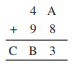Here answer's unit place = 3 , possible addition of unit places digit = 13  A + 8 = 13    A = 13 - 8 = 5 remainder = 1 Ten's place of answer  = 4 + 9 + 1 =  14  B = 4  remainder = 1  100's place = C = 1   Hence value of  A = 5 , B = 4 and C = 1.

Q1. Find the values of the letters in each of the following and give reasons for the steps involved.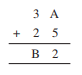Here we are adding two numbers and unit place of the first number and the second number is A and  5 respectively. unit place of the answer is 2 so the way we can get this result is when we get 12 on adding unit places of both number i.e.                                                             A + 5 = 12                                 which implies      A = 12 - 5 = 7. Ten's digit of both...

Q1. If the division N ÷ 2 leaves a remainder of 1, what might be the one’s digit of N? (N is odd; so its one’s digit is odd. Therefore, the one’s digit must be 1, 3, 5, 7 or 9.)

The detailed solution for the above-written question is as follows N is odd; so it's unit digit is odd. Therefore, the unit digit must be 1, 3, 5, 7 or 9.

Q1. If the division N ÷ 5 leaves a remainder of 3, what might be the ones digit of N? (The one’s digit, when divided by 5, must leave a remainder of 3. So the one’s digit must be either 3 or 8.)

The detailed solution for the above-written problem is as follows, The unit digit, when divided by 5, must be leaving a remainder of 3. So the unit digit must be either 3 or 8.

Q2.

(ii) Write a 4-digit number as

If the number is divisible by 11, then what can you say about  ?

If the number abcd is divisible by 11 then [ (b + d) - (a + c) ] also must be divisible by 11. let the number be 1089 here a = 1, b = 0, c = 8 and d = 9 1089 = 1000*1 + 100*0 + 10*8 + 9          = (1001*1 + 99*0 + 11*8) + [(0 + 9) - (1 + 8)]          = 11(91*1 + 9*0 + 8) + [ 9 - 9 ] here  [ (b + d) - (a + c) ] = [9 - 9 ] = 0 which is divisible by 11. hence If the number abcd is divisible by 11...

Q2.

(i) Write a 3-digit number as

If the number is divisible by 11, then what can you say about

Is it necessary that   should be divisible by 11?

let the number abc be 132 Here a = 1, b = 3 and c = 2 132= 100*1 + 10*3 + 2 = 99 + 11*3 + (1 - 3 + 2)     = 11(9*1+3) + (1 - 3 + 2 )  if number is divisible by 11 then (a - b + c )  must be divisible by 11. as in above case of number 132 the a - b + c = 1 -3 + 2 = 0 whichis divisible by 11. Hence we conclude ( a - b + c ) should be divisible by 11 if abc is divisible by 11.

Q1. You have seen that a number 450 is divisible by 10. It is also divisible by 2 and 5 which are factors of 10. Similarly, a number 135 is divisible 9. It is also divisible by 3 which is a factor of 9.

Can you say that if a number is divisible by any number m, then it will also be divisible by each of the factors of m?

Yes, it has been prooved that if a number is divisible by any number m, then it will also be divisible by each of the factors of m. Let's Assume n is divisible by m, and m is divisible by k.This means n=pm and m = qk where all are integers Now, n = p(qk) =( pq)k which means n is divisible by k. Hence a number is divisible by any number m, then it will also be divisible by each of the factors of m.

Check the divisibility of the following numbers by 3.

5. 927

Any number will be divisible by 3 only if the sum of all the digits in that number will be divisible by 3. Sum of digits of number 927 = 9 + 2 + 7 = 18 which is divisible by 3.  Hence we conclude number 108 is divisible by 3.

Check the divisibility of the following numbers by 3.

Q4. 432

Any number will be divisible by 3 only if the sum of all the digits in that number will be divisible by 3. Sum of digits of number 432 = 4 + 3 + 2 = 9 which is divisible by 3.  Hence we conclude number 432 is divisible by 3.

Check the divisibility of the following numbers by 3.

Q3. 294

Any number will be divisible by 3 only if the sum of all the digits in that number will be divisible by 3. Sum of digits of number 294= 2 + 9 + 4 = 15 which is divisible by 3.  Hence we conclude number 294 is divisible by 3.

Check the divisibility of the following numbers by 3.

Q2. 616

Any number will be divisible by 3 only if the sum of all the digits in that number will be divisible by 3. Sum of digits of number 616 = 6 + 1 + 6 = 13 which is not divisible by 3.  Hence we conclude number 616 is not divisible by 3.
Exams
Articles
Questions